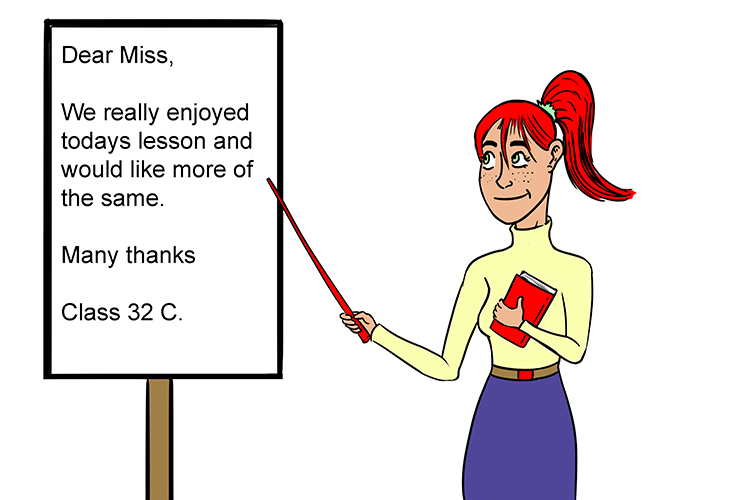# Subject of a formula

Subject of a formula – It is the variable everything is equal to (it’s the letter on its own).Todays subject will be how to write a letter.

Example 1

x=2y+4z

‘x’ is the subject of the formula because it is the LETTER everything is equal to.

Example 2

Rearrange the following equation to make C the subject.

A = BC-3D

Answer: C=(A+3D)/B

Now C is the subject of the formula.